# Geometry P.4

##### This Unit is about Geometry which is studied in Primary Four.

Triangle

A triangle is a three sided polygon.

Types of triangles

1. Right angled trianglesPentagon

It is a five sided figure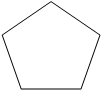SOLID SHAPES.

A solid shape has faces, edges and vertices.

Example

Sphere, cone, cuboid, cube, pyramid, prism, cylinder, etc.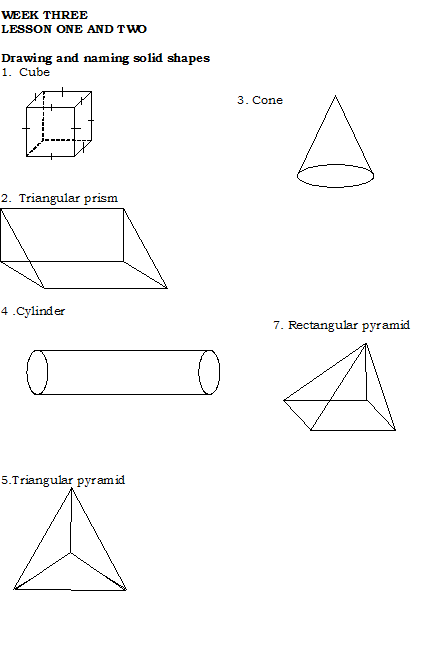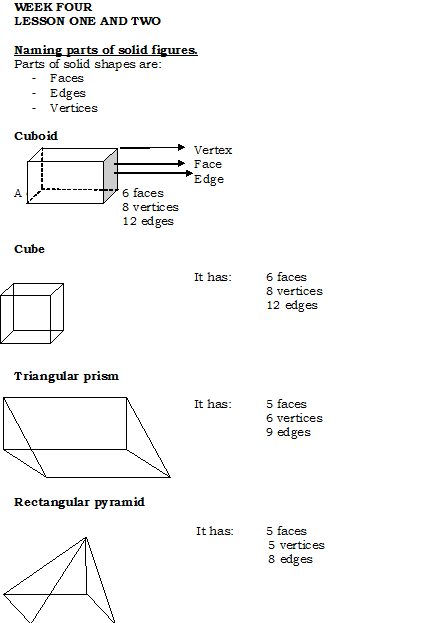Constructing an angle of 90◦

Steps;

• Draw a line of any length
• -mark the centre on the line
• -open the compass to any length
• -stand at the centre and mark equidistant points on either sides
• -stand on each point and mark crossing arcs
• -draw a line to pass through the crossing arcs and the centre.

Finding the missing angles on a right angle.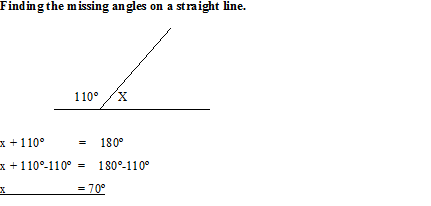SEE ALL
•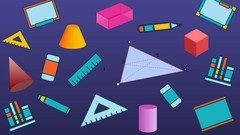YOU